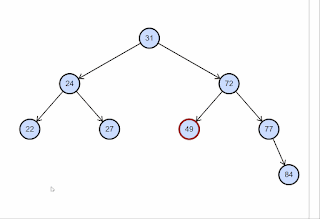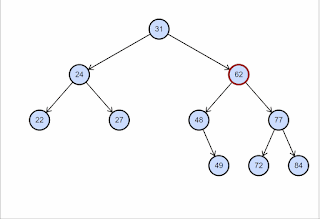# Delete Node In Binary Search Tree -C#

This is the continuation of How to create a binary search tree in c# In this post, I will show you how to remove the node from Binary Search Tree.{alertInfo}

There are three cases.

• The node has no children (it’s a leaf node). You can delete it. In the following tree, I am deleting node 49• The node has just one child. To delete the node, replace it with that child. In the following tree, I am deleting node 48, which has child 49• The node has two children. In this case, you find the deepest node’s value in the rightmost node in the subtree rooted by the left child. That node will not have the right child. First, delete it. Then use it to replace the node that you are deleting. In the following tree, I am deleting node 62, which has two children.``````void Main()
{
BinaryTree tree = new BinaryTree();
tree.Insert(4);
tree.Insert(2);
tree.Insert(5);
tree.Insert(1);
tree.Insert(3);
tree.DisplayTree();
tree.Remove(4);
Console.WriteLine();
tree.DisplayTree();
}

public class Node
{
public int Data { get; set; }
public Node Left { get; set; }
public Node Right { get; set; }

public Node(int data)
{
this.Data = data;
Left = null;
Right = null;

}
}
public class BinaryTree
{
private Node _root;
public BinaryTree()
{
_root = null;
}
public void Insert(int data)
{
// 1. If the tree is empty, return a new, single node
if (_root == null)
{
_root = new Node(data);
return;
}
// 2. Otherwise, recur down the tree
InsertRec(_root, new Node(data));
}
private void InsertRec(Node root, Node newNode)
{
if (root == null)
root = newNode;

if (newNode.Data < root.Data)
{
if (root.Left == null)
root.Left = newNode;
else
InsertRec(root.Left, newNode);

}
else
{
if (root.Right == null)
root.Right = newNode;
else
InsertRec(root.Right, newNode);
}
}
private void DisplayTree(Node root)
{
if (root == null) return;

DisplayTree(root.Left);
System.Console.Write(root.Data + " ");
DisplayTree(root.Right);
}
public void DisplayTree()
{
DisplayTree(_root);
}
public void Remove(int key)
{
RemoveHelper(_root, key);
}

private Node RemoveHelper(Node root, int key)
{

if (root == null)
return root;
//if key is less than the root node,recurse left subtree
if (key < root.Data)
root.Left = RemoveHelper(root.Left, key);
// if key is more than the root node,recurse right subtree
else if (key > root.Data)
{
root.Right = RemoveHelper(root.Right, key);
}

//else we found the key
else
{
//case 1: Node to be deleted has no children
if (root.Left == null && root.Right == null)
{
//update root to null
root = null;
}
//case 2 : node to be deleted has two children
else if (root.Left != null && root.Right != null)
{
var maxNode = FindMax(root.Right);
//copy the value
root.Data = maxNode.Data;
root.Right = RemoveHelper(root.Right, maxNode.Data);
}
//node to be deleted has one children
else
{
var child = root.Left != null ? root.Left : root.Right;
root = child;
}

}

return root;

}
private Node FindMax(Node node)
{
while (node.Left != null)
{
node = node.Left;
}
return node;
}
}

``````